# Anomalous measurements: recent LHCb results deepen ﬂavour puzzle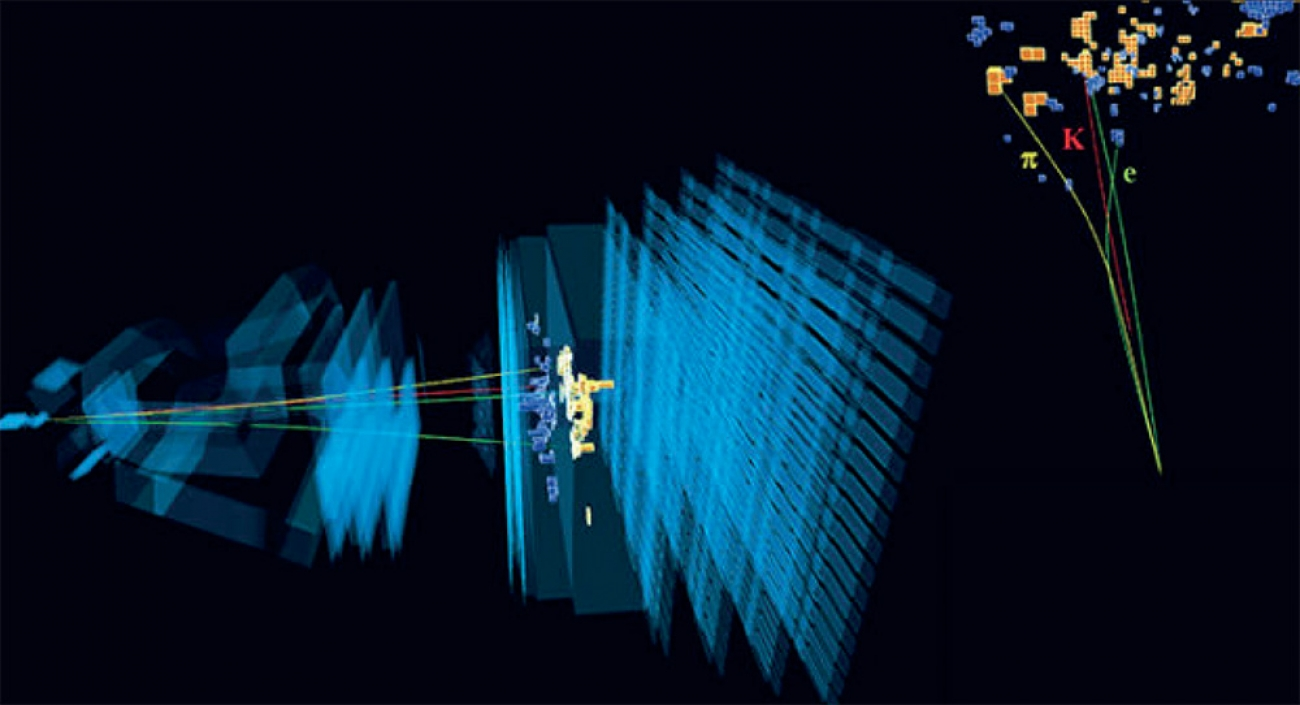The crown jewel of particle physics, the Standard Model (SM), has withstood numerous experimental trials. However, there are still some observations it cannot explain. Examples such as dark matter and the matter-antimatter imbalance in the Universe come to mind. The SM may be extended, by including additional particles and interactions, so as to explain such phenomena. These new particles and interactions are collectively referred to as “new physics” (NP), and the results covered by this article provide a promising lead for their discovery.

### Flavour anomalies

In the past decade, a pattern has been emerging in the study of b-quark decays with leptons in the final state. They are collectively referred to as “flavour anomalies”, and they typically feature tensions at the level of 2–3 standard deviations between experimental results and SM predictions. As such, individual anomalies are not sufficiently significant to claim discovery of NP. However, the anomalies are often treated collectively in an Effective Field Theory (EFT) framework, whereby short distance contributions are separated from their long distance counterparts. This is similar to the 4-point approximation used to describe beta decay, where the process is observed at long enough distances to regard the W boson propagator as point-like.

The EFT approach leads to the formulation of an effective Lagrangian, Leff = Σi CiOi , where large energy-scale effects are encoded in the so-called Wilson coefficients Ci , and small energy-scale contributions are accounted for by the Wilson operators Oi . Given the absence of direct discoveries at the LHC, NP is assumed to be characterised by large (above TeV) energy scales. The anomalous flavour observables could then be impacted indirectly, since NP particles may manifest themselves virtually. From an EFT prespective, this would lead to values of the Ci coefficients that differ from the SM values.

Two examples of EFT analyses of flavour anomalies are shown in Figure 1 [1-3]. In the plot on the left-hand side, the 1σ and 2σ confidence levels for the best-fit point for the considered anomalies are shown in red. On the right-hand side, the best-fit 1σ, 2σ, and 3σ confidence levels are shown in green. The two analyses make different assumptions on the presence of NP in the coefficients Ci (as shown by the axes), however they both find that the flavour anomalies prefer Ci values that are significantly different from the ones predicted by the SM (the origin in each plot).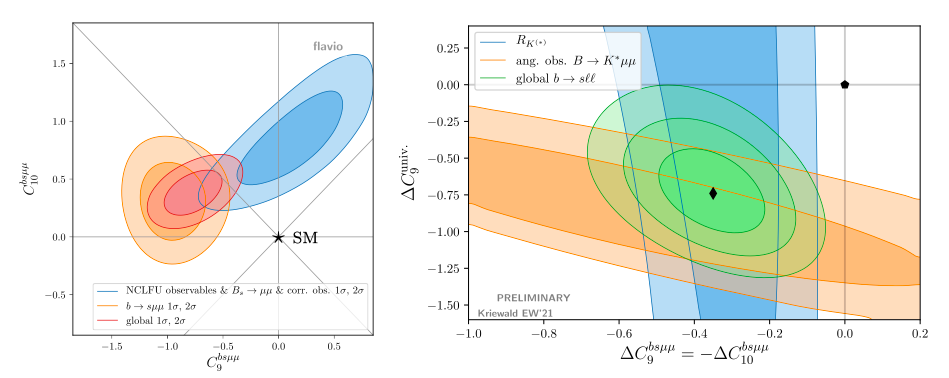### Clean observables

There are several types of anomalous flavour observables, such as differential branching fractions and angular coefficients. They are predicted in the SM with various degrees of precision, and so particularly important are the flavour anomalies that are theoretically clean. Recently, at the Electroweak session of the Moriond 2021 conference, the LHCb collaboration has presented the most precise individual measurements to date of two such theoretically-clean anomalous observables. The first one is the ratio of branching fractions RK = B(B±K±μ+μ) / B(B±K±e+e) . The second one is the branching fraction of Bs0μ+μ , including a search for B0μ+μ and Bs0μ+μγ [5,6]. The processes involved are examples of Flavour Changing Neutral Currents (FCNCs), which are forbidden at tree-level in the SM. As a result, the leading-order Feynman diagrams contain loops, as exemplified by Figure 2. The loops introduce additional vertices that make these processes rare.

The branching fraction of Bs0μ+μ is predicted by the SM with subpercent precision, thanks to the purely-leptonic final state: (Bs0μ+μ) = (3.66 ± 0.14) × 10−9 . The two B±K±+ processes upon which RK is built have branching fractions of O(10−6) . They differ only by the leptons in the final state, which means RK can be predicted accurately by virtue of Lepton Flavour Universality (LFU). This is an accidental symmetry of the SM, whereby the different lepton flavours couple in the same way to vector bosons. As a result, RK is predicted to be approximately 1, with small deviations induced by phase space differences and QED corrections [9,10].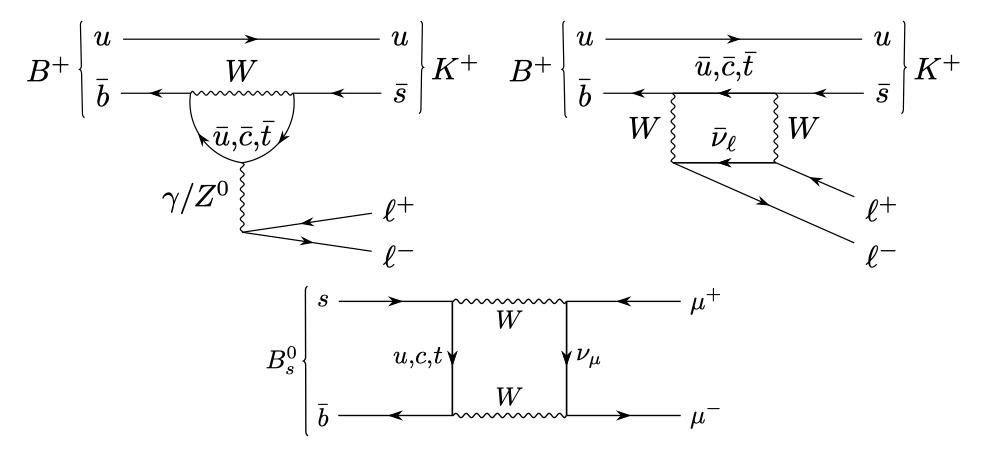##### Figure 2: Examples of lowest-order Feynman diagrams allowed in the SM for B+ → K+ℓ+ℓ− (top) and Bs0 → μ+μ− (bottom). Since FCNCs are forbidden at tree-level, each of these diagrams contains a loop.

Despite the unambiguous SM predictions, experimental results on B(s) μ+μand RK were exhibiting tensions above 2σ before the Moriond 2021 conference, as shown in Figure 3. The LHCb measurements shown here used 5 fb−1 of proton-proton collision data, corresponding to approximately half of the currently available dataset. Given the bigger picture in the context of the flavour anomalies, updates to B(s) μ+μand RK based on the full LHCb data set are crucial to better understanding whether there is NP in rare b-decays.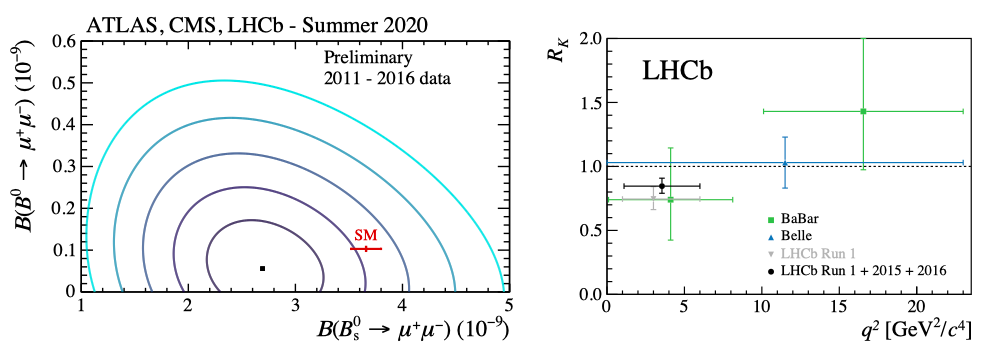##### Figure 3: Experimental status of Bs0 → μ+μ− (left) and RK (right) before the Moriond 2021 conference. Shown on the left are 1–5σ confidence regions (shades of blue) for the combination  of Bs0 → μ+μ− and B0 → μ+μ− measurements from ATLAS , CMS , and LHCb , alongside the SM prediction (red). Shown on the right are results on RK from LHCb [15,16] (black and grey), BaBar  (green), and Belle  (blue); the latter has since been updated . The SM prediction is shown as a dashed line at RK = 1.

Measurement of RK

Highly anticipated updates to the RK and B(s) μ+μmeasurements, now including the full dataset of 9 fb−1 of proton-proton collisions collected by LHCb, were presented at the Moriond 2021 conference. At the core of the RK measurement is the comparison of selection efficiencies between electrons and muons. The masses of the two leptons differ by two orders of mangitude, which leads to them interacting differently with the detector. Muons traverse the LHCb detector almost undisturbed, before finally being stopped by the muon stations. However, electrons are subject to significant loss of energy to bremsstrahlung radiation, leading to worse momentum and mass resolution compared to muons. In order to suppress detection effects that are systematically different between the two lepton flavours, RK is measured as a double ratio: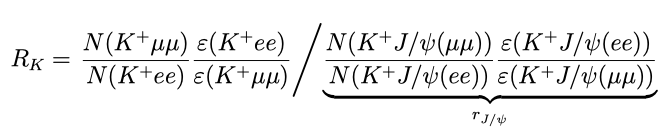where N(X) and ε(X) represent respectively the yields and efficiencies of selecting the decay of a B+ meson into X. The J/ψ modes upon which the single ratio rJ/ψ is built are used to calibrate efficiencies and conduct cross-checks. These modes are characterised by high statistics, and are known to respect LFU . In addition, the B±K±J/ψ (+) (control) channels are kinematically similar to the B±K±+ (rare) channels, and so the data are selected with identical requirements for both. The only exceptions are the cut on the dilepton invariant mass squared, q2, and the reconstructed B mass. The control data are selected using a q2 window around the J/ψ resonance, whilst the B±K±+ candidates are required to have q2 between 1.1 and 6.0 GeV2. This q2 window is chosen to prevent contamination from resonances such as φ(1020) (at low q2) and J/ψ (at high q2).

Several cross-checks are performed to ensure the selection efficiencies are well understood. Among these checks are the ratios rJ/ψ and Rψ(2S) . Like RK , the latter is a double ratio with respect to rJ/ψ, the difference being that the numerator comes from the ψ(2S) resonance. The ratios are found to be rJ/ψ = 0.981 ± 0.020 (stat + syst), and Rψ(2S) = 0.997 ± 0.011 (stat + syst). Both are compatible with the LFU expectation of 1. This confirms that the efficiencies are valid across q2, and that systematic effects successfully cancel out in the double ratio.

The value of RK is extracted from an unbinned maximum likelihood fit to the selected     B±K±e+e and B±K±μ+μ data. The different mass resolutions lead to different background components in the fit model, as shown in Figure 4. The result for RK  is: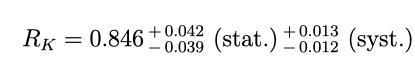As expected, the uncertainty on the result is dominated by the statistical component, rather than the systematic one. The dominant systematic effect is the choice of the fit model, which contributes by around 1%. By comparison, effects induced by the calculation of efficiencies are reduced to the permille level by the double ratio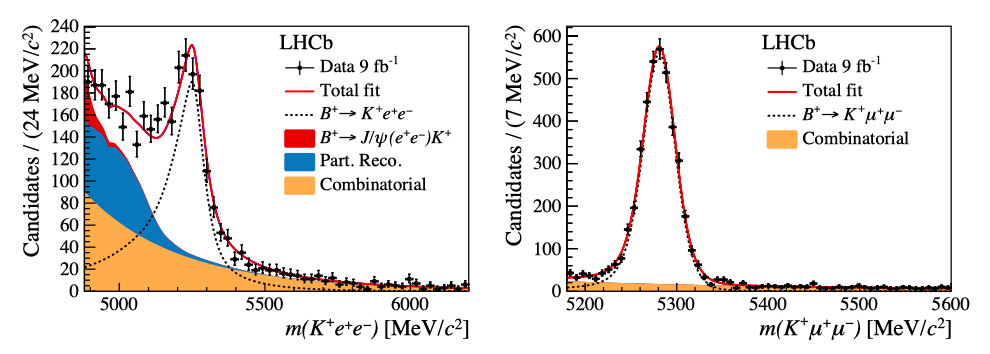### Branching fractions of B(s)0 → µ+µ−(γ)

Thanks to the excellent mass resolution of muons, the signature of B(s) μ+μ decays is very clean. It consists of a pair of oppositely charged muons that have an invariant mass around mass of the B(s) , and that form a vertex that’s significantly displaced from the interaction point. The branching fraction of the signal decays are extracted from an unbinned maximum likelihood fit to the dimuon invariant mass and are measured relatively to two normalisation channels, B±K±J/ψ (μ+μ) and B0K+π which both share similarities with the signal. The former is characterised by similar particle identification and trigger performance, and the latter has similar kinematics. The branching fraction is written as: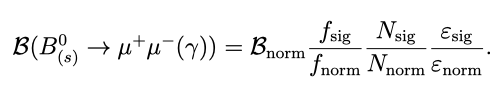where Nsig and Nnorm are the yields of the signal and normalisation channels, respectively. The corresponding efficiencies and fragmentation fractions are denoted by εnorm(sig) and fnorm(sig) respectively. LHCb provided a measurement for the latter in . In order to maximise sensitivity to the signal, a boosted decision tree (BDT) is used to separate signal and background

The fit for the B(s) μ+μ yields is performed simultaneously on subsets of the data separated by BDT output. The left-hand side plot of Figure 5 shows the expected relative yield of B(s) μ+μ,in bins of BDT score, as estimated using two different methods. The red distribution uses a BDT calibration based on the control channel B0K+π, as done in the previous LHCb analysis . The black histogram uses calibrated B(s) μ+μ simulation. The two methods yield compatible results, and the new BDT calibration method significantly improves the precision on the expected relative yield. This is important because it directly improves the total uncertainty of the branching fraction measurement.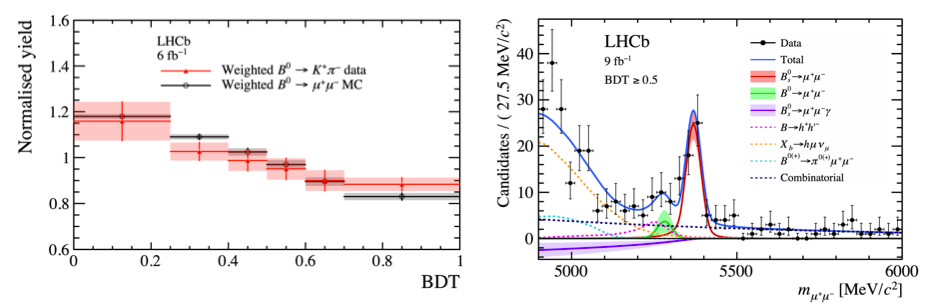##### Figure 5: Left: expected distribution of the relative signal yield, as a function of the BDT score. Right: invariant-mass distribution of B(s) → μ+μ− candidates with BDT score above 0.5. The total fit model is shown in blue, alongside the individual components that represent signal and background.

The branching fraction of B(s) μ+μ is obtained from a fit to the invariant mass of the dimuon system, which is shown on the right-hand side of Figure 5. In addition to the Bs0μ+μsignal, the fit model contains contributions from B0μ+μand Bs0μ+μγ. These two components are compatible with the background-only hypothesis, and so upper limits are set on their corresponding branching fractions. In summary, the results are: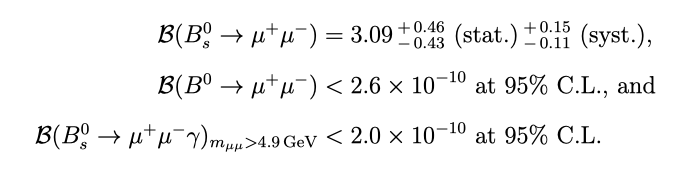Like RK, the measurement of B(Bs0μ+μ) is dominated by statistics. The systematic uncertainty is largely due to the uncertainty on fs/fd. The measured values are compatible with the previous results, as well as with the SM predictions, at the 1σ level.

### Status and prospects

The updaded results on RK and the B(s) μ+μ branching fractions are summarised in Figure 6. While the SM prediction for B(B(s) μ+μ) still lies within the 95% C.L. of the experimental result, the central value is relatively unchanged with respect to the previous LHCb measurement. The same is true for the central value of the RK result: thanks to the increase in statistics with respect to the previous measurement, it now exhibits 3.1σ tension with respect to the value predicted by the SM [9,10]. Therefore, this RK measurement represents the first evidence for the violation of LFU in B±K±+ decays.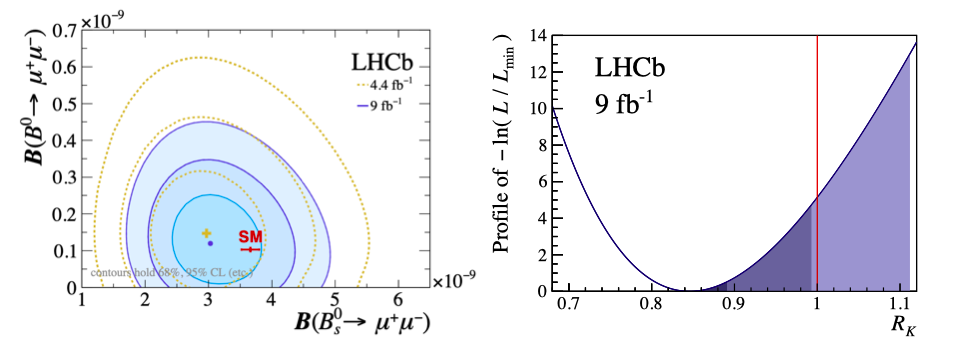##### Figure 6: Experimental status of B(s) → μ+μ− (left) and RK (right) after the Moriond 2021 conference. Shown on the left are 1–3σ confidence regions (shades of blue) of Bs0→ μ+μ− and B0→ μ+μ− measurements from LHCb [5,6], alongside the SM prediction (red). Shown on the right is the likelihood function from the fit to B± → K±ℓ+ℓ− candidates, profiled in RK . The extent of the dark, medium and light blue regions show the values allowed for RK at the 1σ, 3σ, and 5σ levels, respectively. The SM prediction is shown as a solid line at   RK = 1.

Since all presented measurements are dominated by the statistical uncertainty, future updates are crucial to the better understanding of the flavour puzzle. In Table 1 are summarised the projected improvements on the precision of the measurements of RK and B(s) μ+μ the following years of data taking. The limiting factor in the precision of RK is the yield of the electron mode, which, assuming the same detector performance as in    Run 1, would increase more than 40 times at the end of Run 4. This would bring the statistical uncertainty on RK on par with the QED corrections. The error projection on   Bs0μ+μ at future upgrades depends on the assumptions made on the systematic uncertainties, particularly fs/fd . A conservative estimate of 4% would imply an uncertainty of B(Bs0μ+μ) to be approximately 0.30 × 10−9 with 23 fb−1 and 0.16 × 10−9 with    300 fb−1 of data .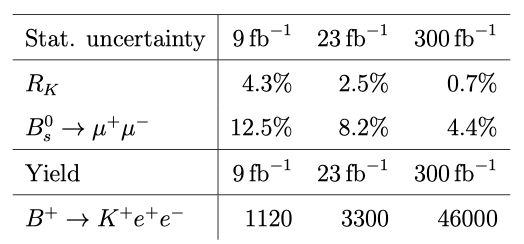Table 1: Extrapolation, based on Run 1 results, of statistical uncertainties on RK, B(Bs0μ+μ) (expressed in units of their SM predictions), and the expected electron-mode yield at the end of future detector upgrades. The b-quark production cross-section is assumed to scale linearly with centre-of-mass energy, and the detector performance is assumed to be unchanged with respect to Run 1. Adapted from Ref. .

The EFT interpretation in terms of shifts in muonic Wilson Coefficients ∆Ciμ from their SM value, in light of the new results of RK and B(s) μ+μ , is updated in Figure 7 . In the  plot on the left, the light orange horizontal band highlights the interval of ∆C10μpreferred by the combined ATLAS, CMS, and updated LHCb measurements of B(B(s) μ+μ). The elongated purple shape marks the regions of ∆C9μ and ∆C10μcompatible with the measured values of RK and RK* . Thanks to their different sensitivity to the ∆Ciμ the combination of these two measurement forms the unfilled contours, which are significantly displaced from the SM point. In the plot on the right, the contribution from other b±s±+ observables, such as the angular ones in the BK*μ+μ system , is included in the ∆Ci fit. While the clean observables presented in this article are insensitive to a flavour-universal shift in C9 , ∆C9U, the observables characterised by less precise theoretical predictions are nonetheless able to provide substantial sensitivity to potential shifts in the muonic Wilson coefficients, when combined with RK and B(s) μ+μ.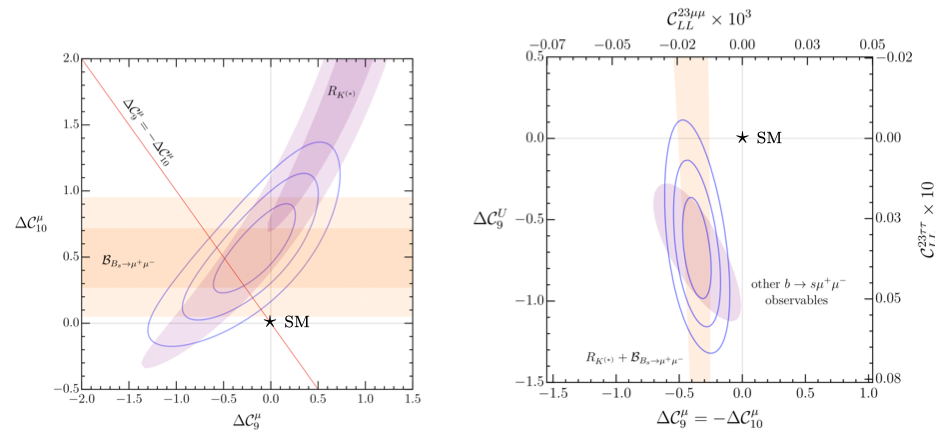##### Figure 7: EFT constraints from the b± → s±ℓ+ℓ− anomalies . Left: Results of the  two-dimensional fit to muonic ∆C9μ and ∆C10μusing clean observables only (1σ, 2σ and 3σ intervals). Also shown are the 1σ and 2σ intervals from RK , RK* , and Bs0→ μ+μ− , the latter under the hypothesis ∆C9U = 0. Right: Results of the two-dimensional fit to ∆C10μ = −∆C9μ and universal ∆C9U using all b± → s±ℓ+ℓ− observables. The vertical band shows the result using clean observables only (1σ interval), while the ellipse denotes the contribution of all the other observables, estimated using Flavio  (1σ interval).

In light of the recent results from LHCb, the flavour puzzle has become even more intriguing. LHCb will continue to improve the precision on its anomalous flavour measurements, whilst also investigating related observables that could provide complementary information. Verification from other experiments, such as Belle II, is expected in the near future, so exciting times lay ahead of the particle physics community!

 W. Altmannshofer and P. Stangl, New Physics in Rare B Decays after Moriond 2021, arXiv:2103.13370.1

  M. Algueró et al., b → sll global fits after Moriond 2021 results, in 55th Rencontres de Moriond on QCD and High Energy Interactions, arXiv:2104.08921.

 C. Hati, J. Kriewald, J. Orloff, and A. M. Teixeira, The fate of vector leptoquarks: the impact of future flavour data, arXiv:2012.05883.

 LHCb collaboration, R. Aaij et al., Test of lepton universality in beauty-quark decays, arXiv:2103.11769, Submitted to Nature Physics.

 LHCb collaboration, R. Aaij et al., Analysis of neutral B-meson decays into two muons, arXiv:2108.09284, submitted to PRL.

 LHCb collaboration, R. Aaij et al., Measurement of the Bs0→ μ+μ  decay properties and search for the B0μ+μ and Bs0μ+μγ decays, arXiv:2108.09283, submitted to PRD.2021-008.

 M. Beneke, C. Bobeth, and R. Szafron, Power-enhanced leading-logarithmic QED corrections to Bqμ+μ , JHEP 10 (2019) 232, arXiv:1908.07011.

 Particle Data Group, P. A. Zyla et al., Review of Particle Physics, PTEP 2020 (2020), no. 8 083C01.

 M. Bordone, G. Isidori, and A. Pattori, On the Standard Model predictions for R K and R K ∗ , Eur. Phys. J. C76 (2016), no. 8 440, arXiv:1605.07633.

 G. Isidori, S. Nabeebaccus, and R. Zwicky, QED corrections in B±K±+at the double-differential level, JHEP 12 (2020) 104, arXiv:2009.00929.

 LHCb collaboration, Combination of the ATLAS, CMS and LHCb results on the  B(s) μ+μdecays, LHCb-CONF-2020-002. ATLAS-CONF-2020-049, CMS PAS  BPH-20-003, LHCb-CONF-2020-002.

 ATLAS, M. Aaboud et al., Study of the rare decays of Bs0 and B0 mesons into muon pairs using data collected during 2015 and 2016 with the ATLAS detector, JHEP 04 (2019) 098, arXiv:1812.03017.

 CMS, A. M. Sirunyan et al., Measurement of properties of Bs0μ+μ decays and search for B0μ+μ with the CMS experiment, JHEP 04 (2020) 188, arXiv:1910.12127.

 LHCb collaboration, R. Aaij et al., Measurement of the Bs0μ+μ branching fraction and effective lifetime and search for B0μ+μ decays, Phys. Rev. Lett. 118 (2017) 191801, arXiv:1703.05747.

 LHCb collaboration, R. Aaij et al., Search for lepton-universality violation in B±K±+ decays, Phys. Rev. Lett. 122 (2019) 191801, arXiv:1903.09252.

 LHCb collaboration, R. Aaij et al., Test of lepton universality using B±K±+decays, Phys. Rev. Lett. 113 (2014) 151601, arXiv:1406.6482.

 BaBar Collaboration, Measurement of Branching Fractions and Rate Asymmetries in the Rare Decays BK(*)+, Phys. Rev. D86 (2012) 032012.

 Belle collaboration, Measurement of the Differential Branching Fraction and Forward-Backward Asymmetry for BK(*)+, Phys. Rev. D103 (2009) 12.

 Belle, S. Choudhury et al., Test of lepton flavor universality and search for lepton flavor violation in BKℓℓ decays, JHEP 03 (2021) 105, arXiv:1908.01848.

 LHCb, R. Aaij et al., Precise measurement of the fs/fd ratio of fragmentation fractions and of Bs0 decay branching fractions, arXiv:2103.06810.

 LHCb collaboration, Physics case for an LHCb Upgrade II — Opportunities in flavour physics, and beyond, in the HL-LHC era, arXiv:1808.08865.

 C. Cornella et al., Reading the footprints of the B-meson flavor anomalies, arXiv:2103.16558.

 LHCb, R. Aaij et al., Test of lepton universality with B0K*0+ decays, JHEP 08 (2017) 055, arXiv:1705.05802.

 LHCb, R. Aaij et al., Measurement of CP -Averaged Observables in the B0K∗0μ+μ− Decay, Phys. Rev. Lett. 125 (2020), no. 1 011802, arXiv:2003.04831.

 D. M. Straub, Fllavio: a Python package for flavour and precision phenomenology in the Standard Model and beyond, arXiv:1810.08132.